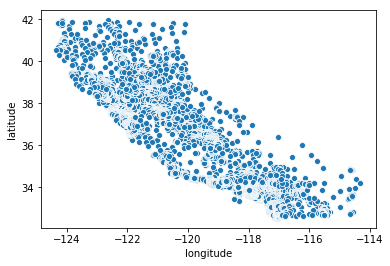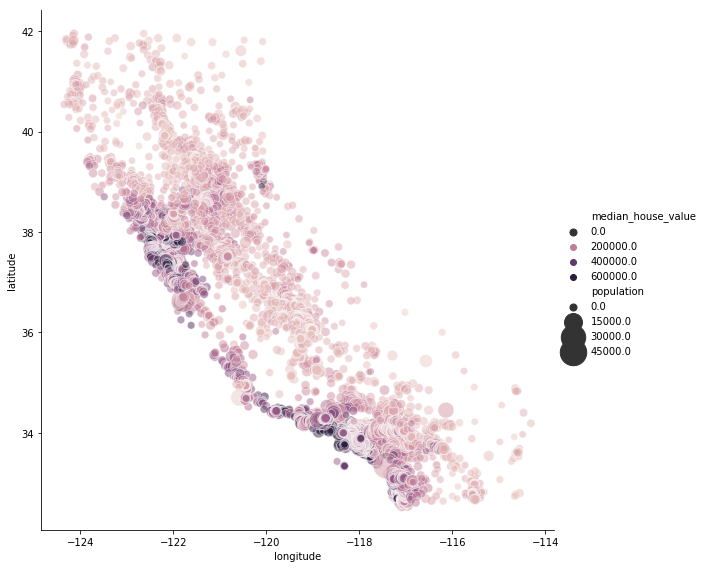# California Housing Prices

Prediction of Median house prices for California districts derived from the 1990 census.

## Context

This is the dataset used in the second chapter of Aurélien Géron’s recent book ‘Hands-On Machine learning with Scikit-Learn and TensorFlow’. It serves as an excellent introduction to implementing machine learning algorithms because it requires rudimentary data cleaning, has an easily understandable list of variables and sits at an optimal size between being to toyish and too cumbersome.

The data contains information from the 1990 California census. So although it may not help you with predicting current housing prices like the Zillow Zestimate dataset, it does provide an accessible introductory dataset for teaching people about the basics of machine learning.

## Acknowledgements

Please refer to the Kaggle challenge web page

## Inspiration

predict a real estate price

# Exploratory Data Analysis

``````import pandas as pd
import numpy as np
import matplotlib.pyplot as plt
import seaborn as sns
import os
``````
``````import folium
``````
``````from sklearn.model_selection import train_test_split
from sklearn.metrics import mean_squared_error
from sklearn.linear_model import Lasso, LinearRegression, Ridge, RANSACRegressor, SGDRegressor
from sklearn.svm import SVR
``````
``````file_path = os.path.join('input', 'house_big.csv')
``````
longitude latitude housing_median_age total_rooms total_bedrooms population households median_income median_house_value ocean_proximity
0 -122.23 37.88 41.0 880.0 129.0 322.0 126.0 8.3252 452600.0 NEAR BAY
1 -122.22 37.86 21.0 7099.0 1106.0 2401.0 1138.0 8.3014 358500.0 NEAR BAY
2 -122.24 37.85 52.0 1467.0 190.0 496.0 177.0 7.2574 352100.0 NEAR BAY
3 -122.25 37.85 52.0 1274.0 235.0 558.0 219.0 5.6431 341300.0 NEAR BAY
4 -122.25 37.85 52.0 1627.0 280.0 565.0 259.0 3.8462 342200.0 NEAR BAY
``````df.shape
``````
``````(20640, 10)
``````

## Content

The data pertains to the houses found in a given California district and some summary stats about them based on the 1990 census data. Be warned the data aren’t cleaned so there are some preprocessing steps required! The columns are as follows, their names are pretty self explanitory:

• longitude
• latitude
• housing_median_age
• total_rooms
• total_bedrooms
• population
• households
• median_income
• median_house_value
• ocean_proximity
``````df.info()
``````
``````<class 'pandas.core.frame.DataFrame'>
RangeIndex: 20640 entries, 0 to 20639
Data columns (total 10 columns):
longitude             20640 non-null float64
latitude              20640 non-null float64
housing_median_age    20640 non-null float64
total_rooms           20640 non-null float64
total_bedrooms        20433 non-null float64
population            20640 non-null float64
households            20640 non-null float64
median_income         20640 non-null float64
median_house_value    20640 non-null float64
ocean_proximity       20640 non-null object
dtypes: float64(9), object(1)
memory usage: 1.6+ MB
``````

There are few missing value int the ‘total_bedrooms’ column. Now let’s see the basic stats for the numerical columns:

``````df.describe()
``````
longitude latitude housing_median_age total_rooms total_bedrooms population households median_income median_house_value
count 20640.000000 20640.000000 20640.000000 20640.000000 20433.000000 20640.000000 20640.000000 20640.000000 20640.000000
mean -119.569704 35.631861 28.639486 2635.763081 537.870553 1425.476744 499.539680 3.870671 206855.816909
std 2.003532 2.135952 12.585558 2181.615252 421.385070 1132.462122 382.329753 1.899822 115395.615874
min -124.350000 32.540000 1.000000 2.000000 1.000000 3.000000 1.000000 0.499900 14999.000000
25% -121.800000 33.930000 18.000000 1447.750000 296.000000 787.000000 280.000000 2.563400 119600.000000
50% -118.490000 34.260000 29.000000 2127.000000 435.000000 1166.000000 409.000000 3.534800 179700.000000
75% -118.010000 37.710000 37.000000 3148.000000 647.000000 1725.000000 605.000000 4.743250 264725.000000
max -114.310000 41.950000 52.000000 39320.000000 6445.000000 35682.000000 6082.000000 15.000100 500001.000000
``````df.ocean_proximity.value_counts()
``````
``````<1H OCEAN     9136
INLAND        6551
NEAR OCEAN    2658
NEAR BAY      2290
ISLAND           5
Name: ocean_proximity, dtype: int64
``````

## Cleaning data

``````df.duplicated().sum()
``````
``````0
``````
``````df.isnull().sum()
``````
``````longitude               0
latitude                0
housing_median_age      0
total_rooms             0
total_bedrooms        207
population              0
households              0
median_income           0
median_house_value      0
ocean_proximity         0
dtype: int64
``````
``````print(f'percentage of missing values: {df.total_bedrooms.isnull().sum() / df.shape * 100 :.2f}%')
``````
``````percentage of missing values: 1.00%
``````
``````df = df.fillna(df.median())
df.isnull().sum()
``````
``````longitude             0
latitude              0
housing_median_age    0
total_rooms           0
total_bedrooms        0
population            0
households            0
median_income         0
median_house_value    0
ocean_proximity       0
dtype: int64
``````

## Dealing with geospatial infos

Visualization of the data in a scatter plot in a “geographic way”

``````sns.scatterplot(df.longitude, df.latitude)
``````
``````<matplotlib.axes._subplots.AxesSubplot at 0x7f244cbecb00>
``````Same plot but this time with a varying size of the data points based on `population` variable and a different color depending of the real estate price (`median_house_value`)

``````sns.relplot(x="longitude", y="latitude", hue="median_house_value", size="population", alpha=.5,\
sizes=(50, 700), data=df, height=8)
plt.show()
````````````# Create a map with folium centered at the mean latitude and longitude
cali_map = folium.Map(location=[35.6, -117], zoom_start=6)

# Display the map
display(cali_map)
``````
``````# Add markers for each rows
for i in range(df.shape):